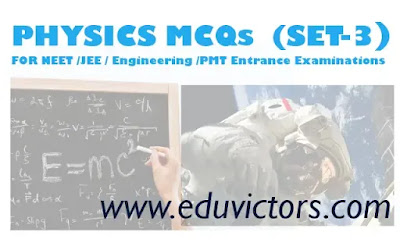## PHYSICS MCQs (Set-3)

FOR JEE NEET Med/Engineering Entrance Examinations

Topics Covered: Kinematics (Motion in a Straight Line)

Q1: A particle starts from rest with uniform acceleration 'a' Its velocity after 'n' second is 'v'. The displacement in last two seconds is:

(a) 2v(n-1)/n
(b) v(n-1)/n
(c) v(n+1)/n
(d) 2v(2n+1)/n

Q2: A body starts from origin and moves along x-axis such that at any instant velocity is v = 4t³ - 2t. The acceleration of the particle when is it is 2m away from the origin is:

(a) 14 m/s²
(b) 18 m/s²
(c) 22 m/s²
(d) 32 m/s²

Q3: If average velocity of particle moving on a straight line is zero in a time interval, then

(a) Acceleration of particle may be zero
(b) Velocity of particle must be zero at an instant
(c) Velocity of particle may be never zero in the interval
(d) Average speed of particle may be zero in the interval

Q4: Two bikes A and B start from a point. A moves with uniform speed 40 m/s and B starts from rest with uniform acceleration 2 m/s². If B starts at t = 0 and A starts from the same point at t = 10s, then the time interval during the journey in which A was ahead of B is:

(a) 20s
(b) 8s
(c) 10s
(d) A is never ahead of B

Q5: If magnitude of average speed and average velocity over an interval of time are same, then

(a) Particle must move with zero acceleration
(b) Particle must move with uniform acceleration
(c) Particle must be at rest
(d) Particle must move in a straight line without turning back

Q6: A point moves with uniform acceleration and v₁, v₂ and v3 denote the average velocities in three successive intervals of time t₁, t₂ and t₃. Which of the following relations is correct:

(a) v₁ – v₂ : v₂ – v3 = t₁ – t₂ : t₂ + t₃
(b) v₁ – v₂ : v₂ – v3 = t₁ + t₂ : t₂ + t₃
(c) v₁ – v₂ : v₂ – v3 = t₁ – t₂ : t1 – t₃
(d) v₁ – v₂ : v₂ – v3 = t₁ – t₂ : t₂ – t₃

Q7: A particle starting with certain initial velocity and uniform acceleration covers a distance of 12 m in first 3 seconds and a distance of 30 m in next 3 seconds. The initial velocity of the particle is

(a) 3 m/s
(b) 2.5 m/s
(c) 2 m/s
(d) 1 m/s

Q8: 100 m and at the same time another ball is projected vertically upwards from ground with a velocity 25 m/s. Then the distance from the top of the tower, at which the two balls meet is

(a) 68.4 m
(b) 48.4 m
(c) 78.4 m
(d) 18.4 m

Q9: A car moving with speed v on a straight road can be stopped with in distance d on applying brakes. If same car is moving with speed 3v and brakes provide half retardation, then car will stop after travelling distance:

(a) 6 d
(b) 3 d
(c) 9 d
(d) 18 d

Q10: Two balls of equal masses are thrown upwards, along the same vertical direction at an interval of  2 seconds, with the same initial velocity of 40 m/s. Then these collide at a height of (Take g=10 /s²):

(a) 120m
(b) 75m
(c) 200 m
(d) 45m

1: (a) 2v(n-1)/n
2: (c) 22 m/s²
3: (b) Velocity of particle must be zero at an instant
4: (d) A is never ahead of B
5: (d) Particle must move in a straight line without turning back
6: (b) v₁ – v₂ : v₂ – v3 = t₁ + t₂ : t₂ + t₃
7: (d) 1 m/s
8: (c) 78.4 m
9: (d) 18 d
10: (b) 75m

Physical Constants
Types of Vectors
Simple Physics Quiz
Physics JEE/NEET/Engineering or PMT Entrance Quiz(SET-1)
Physics JEE/NEET Engineering or PMT Entrance Quiz (Set-2)